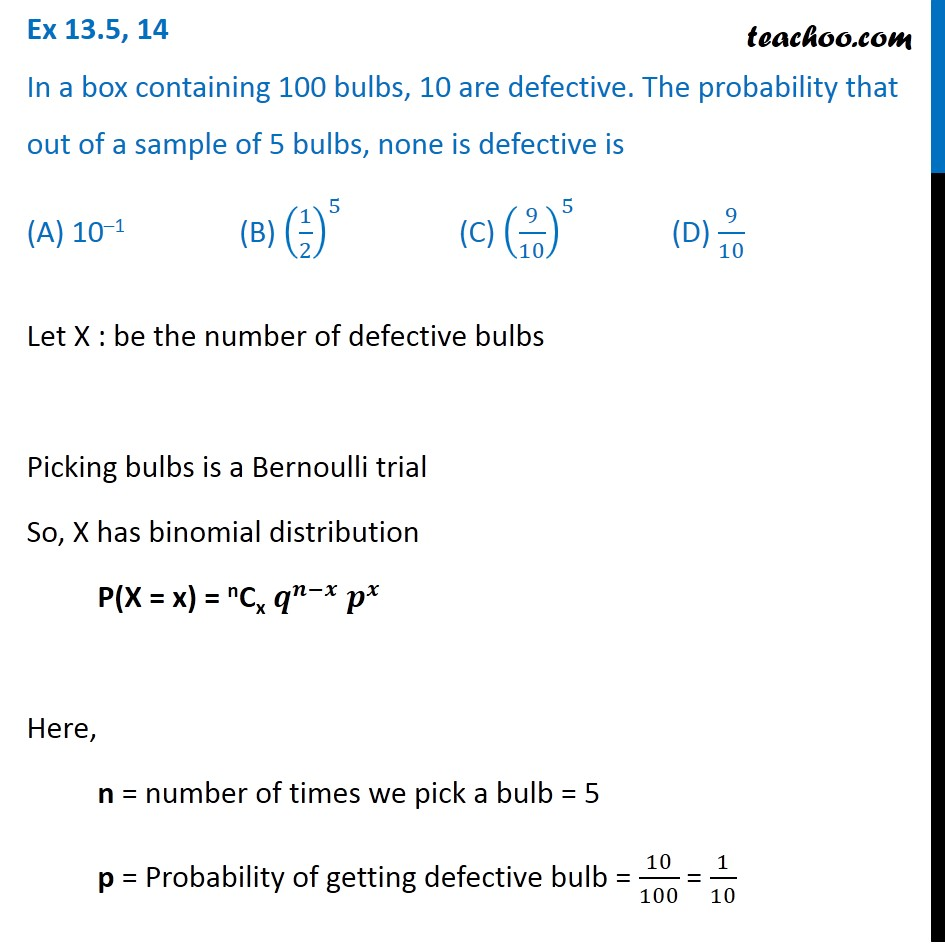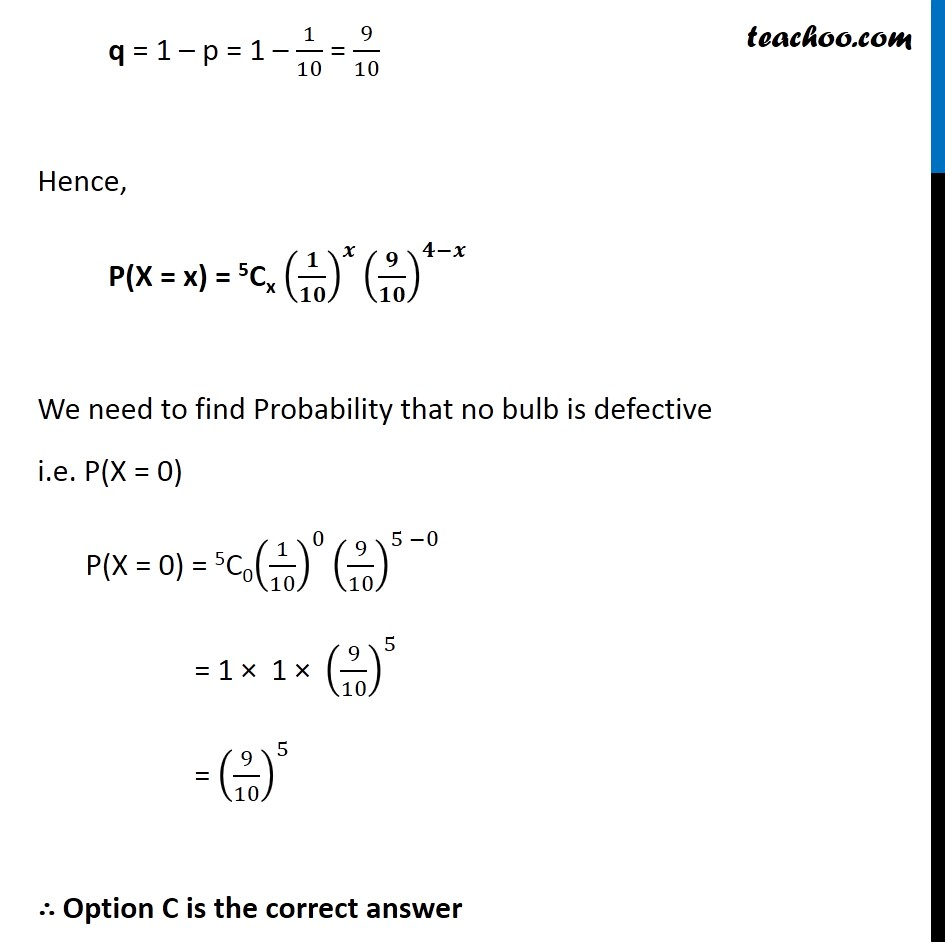Bernoulli Trial

Chapter 13 Class 12 Probability
Serial order wiseLearn in your speed, with individual attention - Teachoo Maths 1-on-1 Class

### Transcript

Question 14 In a box containing 100 bulbs, 10 are defective. The probability that out of a sample of 5 bulbs, none is defective is (A) 10–1 (B) (1/2)^5 (C) (9/10)^5 (D) 9/10aLet X : be the number of defective bulbs Picking bulbs is a Bernoulli trial So, X has binomial distribution P(X = x) = nCx 𝒒^(𝒏−𝒙) 𝒑^𝒙 Here, n = number of times we pick a bulb = 5 p = Probability of getting defective bulb = 10/100 = 1/10 q = 1 – p = 1 – 1/10 = 9/10 Hence, P(X = x) = 5Cx (𝟏/𝟏𝟎)^𝒙 (𝟗/𝟏𝟎)^(𝟒−𝒙) We need to find Probability that no bulb is defective i.e. P(X = 0) P(X = 0) = 5C0(1/10)^0 (9/10)^(5 −0) = 1 × 1 × (9/10)^5 = (9/10)^5 ∴ Option C is the correct answer